Latest Banking jobs   »   Quantitative Aptitude Quiz For RRB PO...   »   Quantitative Aptitude Quiz For RRB PO...

# Quantitative Aptitude Quiz For RRB PO Mains 2021- 10th September

Directions (1-5): In the given questions, two quantities are given, one as ‘Quantity I’ and another as ‘Quantity II’. You have to determine relationship between two quantities and choose the appropriate option:

Q1. Quantity I: A man have two solid balls. Ratio between radius of first ball and second ball is 4 : 3. If man cut the second ball from middle and difference between total surface area of first ball and total surface area of part of second ball is 1424.5 cm². Find value of radius of bigger ball ?

Quantity II: Height of a cylindrical vessel is equal to side of a square having area 256 cm². If volume of cylindrical vessel is 22176 cm. Find the radius of cylindrical vessel.

(a) Quantity I > Quantity II

(b) Quantity I < Quantity II

(c) Quantity I ≥ Quantity II

(d) Quantity I ≤ Quantity II

(e) Quantity I = Quantity II or no relation

Q2.  Quantity I: P alone can do a piece of work in 72 days and Q alone can do same work in 48 days, R is 50% more efficient than Q then find in how many days total work will be completed if all three work on alternate days starting with P and ending with R ?    Quantity II: A alone can do a task in 48 days B is 25% less efficient than A and C can do the same task in 40 days lesser than B does. Then find in how many days all three will complete the task together?

(a) Quantity I > Quantity II

(b) Quantity I < Quantity II

(c) Quantity I ≥ Quantity II

(d) Quantity I ≤ Quantity II

(e) Quantity I = Quantity II or no relation

Q3. Quantity I: A bucket carried one dozen of mangoes. One third of mangoes become bad, if three mangoes taken out from the bucket at random. Then what is probability that one mango out of three mangoes picked up is good?

Quantity II: Find the probability of selecting a face card from a well shuffled pack of card given that 2 additional Joker card also counts as a face card?

(a) Quantity I > Quantity II

(b) Quantity I < Quantity II

(c) Quantity I ≥ Quantity II

(d) Quantity I ≤ Quantity II

(e) Quantity I = Quantity II or no relation

Q4. Quantity I – A train running at the speed of 72 km/hr passed a man in 14 sec and a platform in 32 sec. Find the length of platform ?

Quantity II – A train moves at the speed of 108 km/hr, passes a platform and a bridge in 15 sec and 18 sec respectively. If the length of platform is 50% of length of bridge, then find the length of train.

(a) Quantity I > Quantity II

(b) Quantity I < Quantity II

(c) Quantity I ≥ Quantity II

(d) Quantity I ≤ Quantity II

(e) Quantity I = Quantity II or no relation

Q5. Quantity I: Present age of Randy, if 10 years are subtracted from the present age of Randy, then you would get twelve times of the present age of his grandson Sandy and Sandy is 19 years younger to Sundar whose age is 24.

Quantity II: Average age of the remaining persons in the group if average age of group of 14 persons is 27 years and 9 months. Two persons, each 42 years old, left the group.

(a) Quantity I > Quantity II

(b) Quantity I < Quantity II

(c) Quantity I ≥ Quantity II

(d) Quantity I ≤ Quantity II

(e) Quantity I = Quantity II or No relation

Directions (6-8): In the given questions, two quantities are given, one as ‘Quantity I’ and another as ‘Quantity II’. You have to determine relationship between two quantities and choose the appropriate option:

Q6.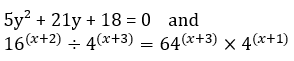Quantity I: Value of y.

Quantity II: Value of x.

(a) Quantity I > Quantity II

(b) Quantity I < Quantity II

(c) Quantity I ≥ Quantity II

(d) Quantity I ≤ Quantity II

(e) Quantity I = Quantity II or no relation

Q7. Quantity I: Find the cost of B per kg if a product C is made of two ingredients A and B in the proportion of 2 : 5. The price of A is three times that of B. The overall cost of C is Rs. 5.20 per kg including labour charges of 80 paisa per kg.

Quantity II: Find the cost of manufacturing each article if a manufacturer estimates that on inspection 12% of the articles he produces will be rejected. He accepts an order to supply 22,000 articles at Rs. 7.50 each. He estimates the profit on his outlay including the manufacturing of rejected articles, to be 20%.

(a) Quantity I > Quantity II

(b) Quantity I < Quantity II

(c) Quantity I ≥ Quantity II

(d) Quantity I ≤ Quantity II

(e) Quantity I = Quantity II or no relation

Q8.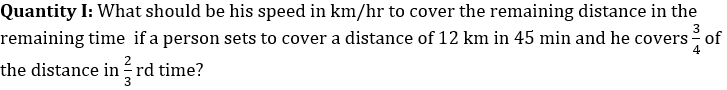Quantity II: What is the speed in km/hr of slower Bus. If two buses, one moving towards North and the other towards South, leave the same place at the same time. The speed of one of them is greater than that of the other by 5 km/h. At the end of 2 hrs, they are at a distance of 50 km from each other?

(a) Quantity I > Quantity II

(b) Quantity I < Quantity II

(c) Quantity I ≥ Quantity II

(d) Quantity I ≤ Quantity II

(e) Quantity I = Quantity II or no relation

Directions (9-10): In the given questions, two quantities are given, one as ‘Quantity I’ and another as ‘Quantity II’. You have to determine relationship between two quantities and choose the appropriate option (compare only magnitudes)

Q9.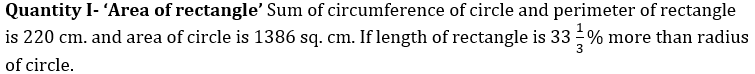Quantity II- ‘Perimeter of square’  Circumference of a circle is 132 cm and area of circle is 710 sq. cm more than area of square

(a) Quantity I > Quantity II

(b) Quantity I < Quantity II

(c) Quantity I ≥ Quantity II

(d) Quantity I ≤ Quantity II

(e) Quantity I = Quantity II or no relation

Q10. Quantity I – Veer takes two times more than the time taken by Sameer to complete a work. Sameer and veer started to work alternatively starting with Sameer on first day and Veer on second day. If both completed work in 36 days, then find in how many days Sameer and Veer will complete the work together, if both work with their double efficiency?

Quantity II – Tap P can fill a swimming pool in 8 days and tap Q can fill the same swimming pool in 24 days, while tap R can empty the swimming pool in 16 days. If all three taps are opened together in swimming pool, then in how much time swimming pool will filled completely?

(a) Quantity I > Quantity II

(b) Quantity I < Quantity II

(c) Quantity I ≥ Quantity II

(d) Quantity I ≤ Quantity II

(e) Quantity I = Quantity II or no relation

Solutions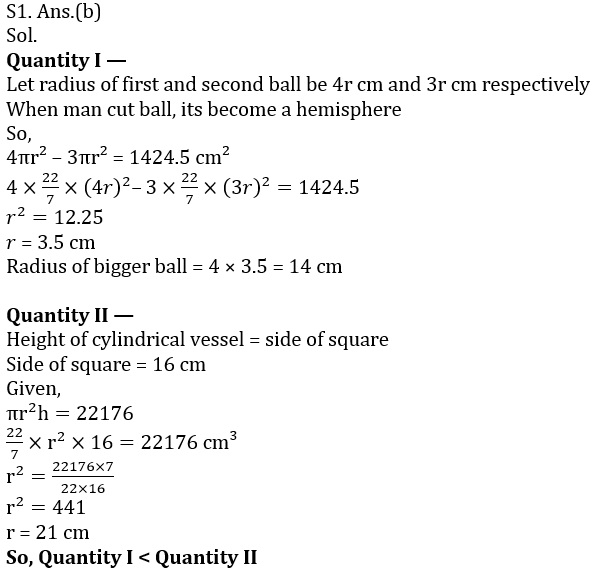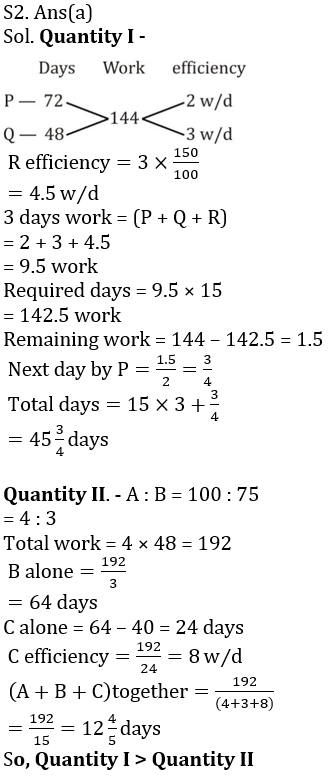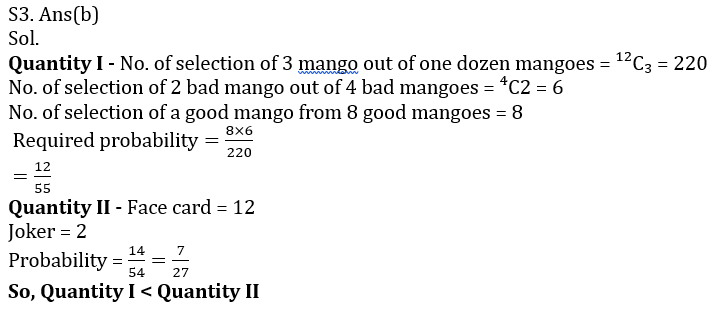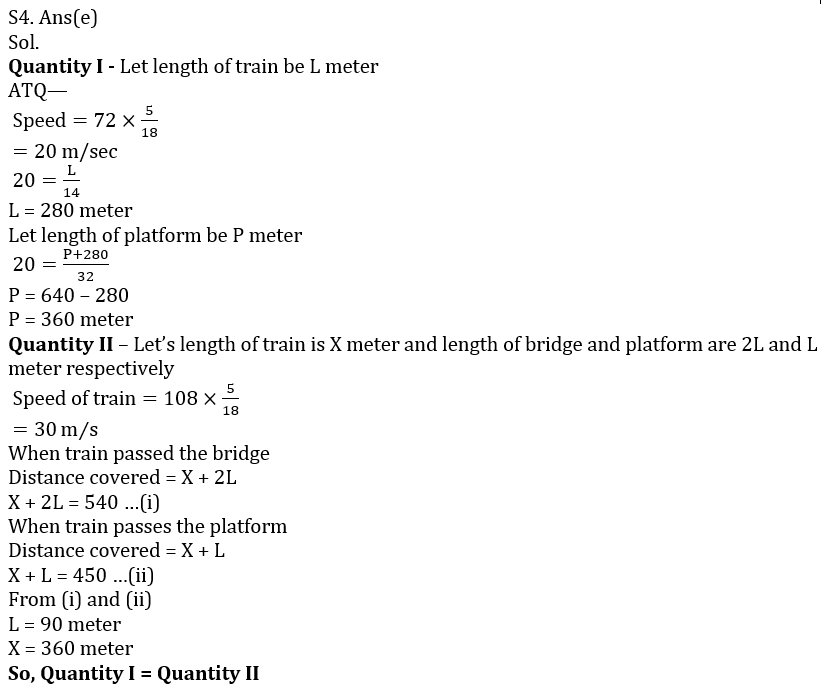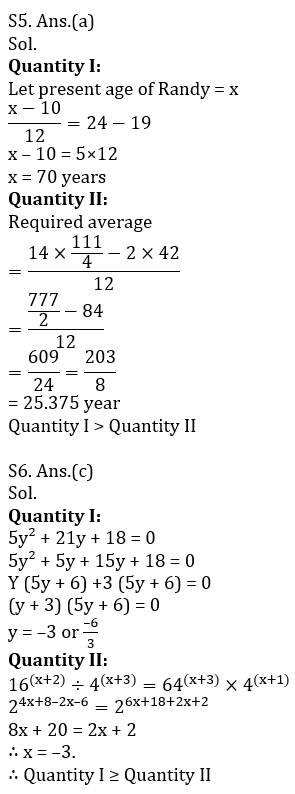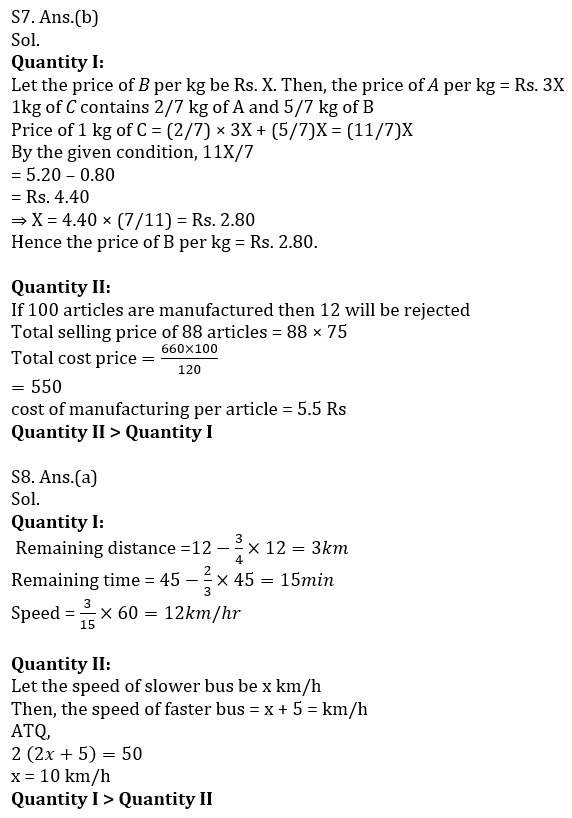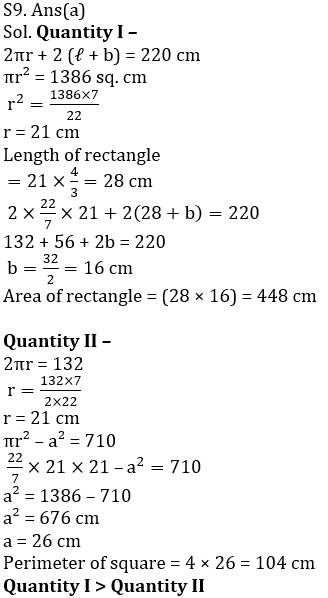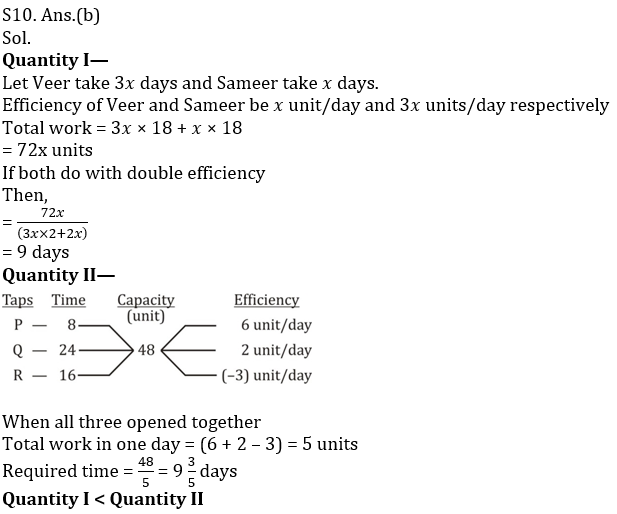Click Here to Register for Bank Exams 2021 Preparation Material#### Congratulations!Download Hindu Review of October 2021: Free PDF